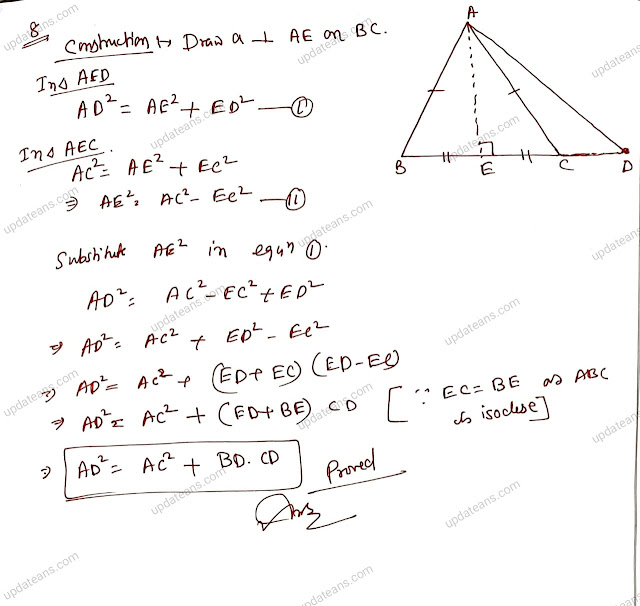## 17 Nov 2023

### Class 9 | Geometry | PQR is a triangle in which angle Q = 2angle R. If a line PS is drawn from vertex P such that it bisects angle QPR and cuts QR at S such that PQ = RS, then what is the value of angle QPR + angle QRP?